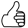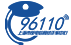• 最近访问：

=IF(B33＜=-10%,"加1000",IF(B33＜=-8%,"加800",IF(B33＜=-6%,"加600",IF(B33＜=-4%,"加400",IF(B33＜=-2%,"加200",IF(B33＞=6%,"开始关注",IF(B33＞=10%,
"赎回盈利",IF(B33＞=50%,"注意全部赎回","不用操作 "))))))))=IF(B33＜=-10%,"加1000",IF(B33＜=-8%,"加800",IF(B33＜=-6%,"加600",IF(B33＜=-4%,"加400",IF(B33＜=-2%,"加200",IF(B33＞=6%,"开始关注",IF(B33＞=10%,
"赎回盈利",IF(B33＞=50%,"注意全部赎回","不用操作 "))))))))